Shipra Saxena — Published On March 12, 2021 and Last Modified On March 18th, 2021

## Objective

• Understand what is edge detection and how it can be helpful in image classification.
• Learn how kernels are used to identify the edges in a given image.

Note: If you are more interested in learning concepts in an Audio-Visual format, We have this entire article explained in the video below. If not, you may continue reading.

## Introduction

Let’s start with an example. Suppose we have given a task to classify a set of images in Cars, Animals, and Humans. Here is a bunch of images. Can you differentiate between the objects? Quite simple, right?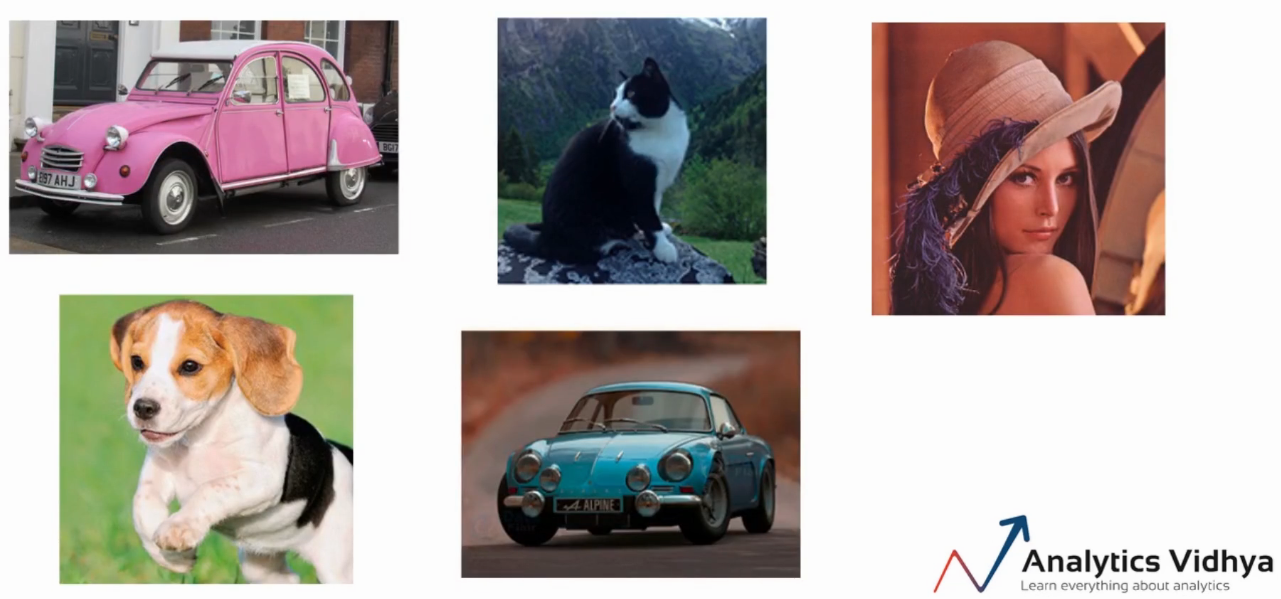Yes, we can easily identify the cars, animals and the human in the above pictures. Now let’s consider another set of images as shown below.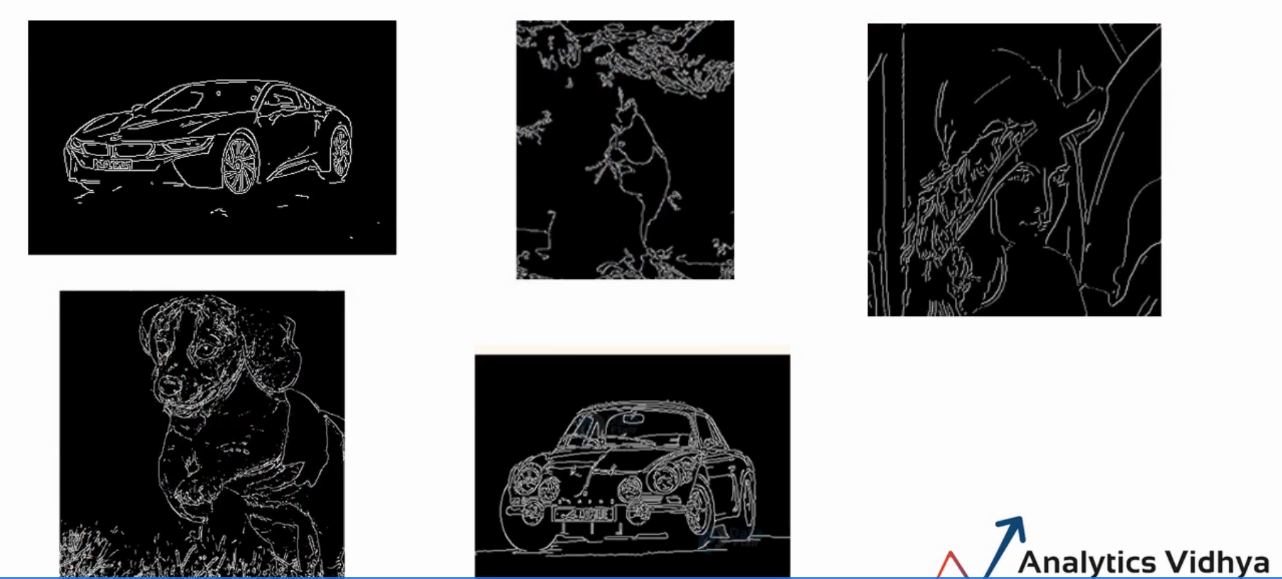Can you still easily classify the images? I believe, yes, we can clearly see there are two cars, two animals and a person.

But, what is the difference between these two sets of images? well, in the second case we removed the color, the background, and the other minute details from the pictures. We only have the edges, and you still able to identify the objects in the image.

So for any given image, if we are able to extract only the edges and remove the noise from the image, we would still be able to classify the image.

## What is Edge Detection?

As we know, the computer sees the images in the form of matrices. As shown here.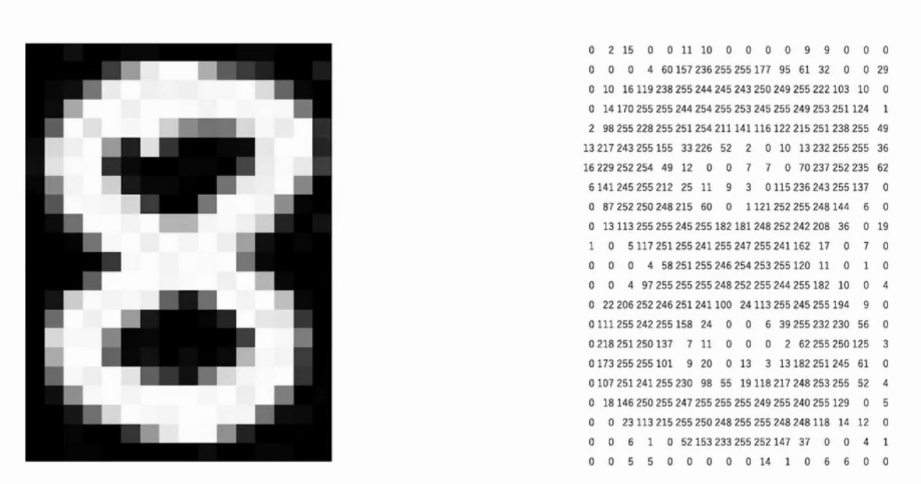In this case, we can clearly identify the edges by looking at the numbers or the pixel values. So if you look closely in the matrix of the numbers, there is a significant difference between the pixel values around the edge. The black area in the left image is represented by low values as shown in the second image. Similarly, the white area is represented by the larger numbers.

Edge detection is an image processing technique for finding the boundaries of an object in the given image.

So, to summarize, the edges are the part of the image that represents the boundary or the shape of the object in the image. Also, the pixel values around the edge show a significant difference or a sudden change in the pixel values.

Based on this fact we can identify which pixels represent the edge or which pixel lie on the edge.

## How to Extract the Edges From An Image?

Once we have the idea of the edges, now let’s understand how can we extract the edges from an image. Say, we take a small part of the image. We can compare the pixel values with its surrounding pixels, to find out if a particular pixel lies on the edge.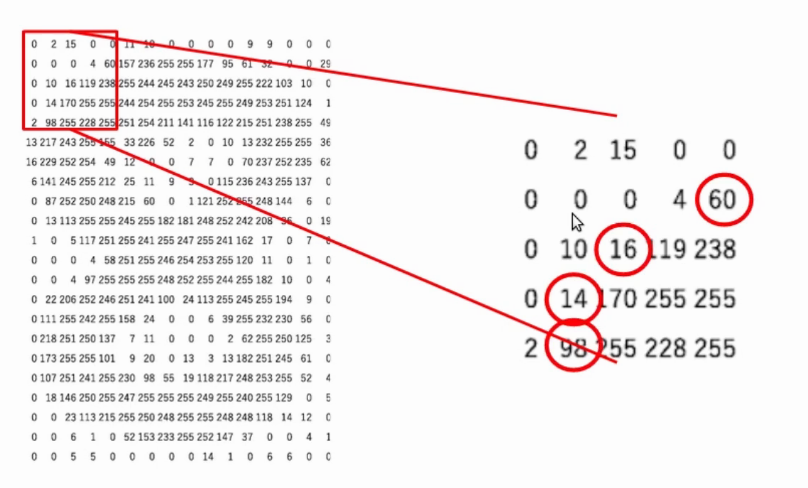For example, if I take the target pixel 16 and compare the values at its left and right. Here the values are 10 and 119 respectively. Clearly, there is a significant change in the pixel values. So, we can say the pixel lies on the edge.

Whereas, if you look at the pixels in the following image. The pixel values to the left and the right of the selected pixel don’t have a significant difference. Hence we can say that this pixel is not at the edge.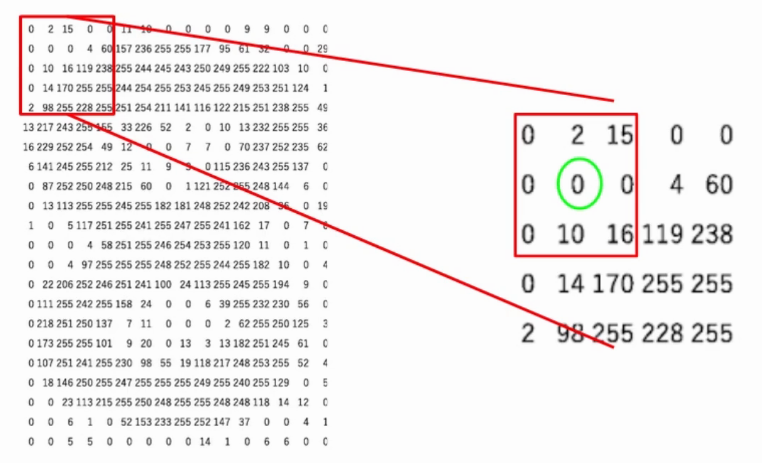Now the question is do we have to sit and manually compare these values to find the edges. Well, obviously not. For the task, we can use a matrix known as the kernel and perform the element-wise multiplication.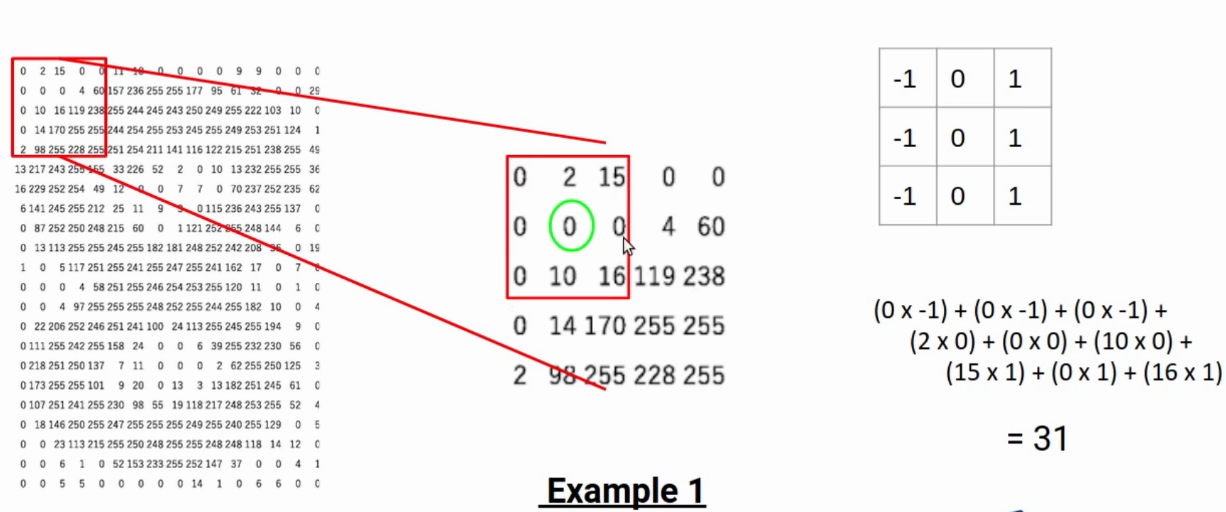Let’s say, in the selected portion of the image, I multiply all the numbers on left with -1, all the numbers on right with 1. Also all the numbers in the middle row with 0. In simple terms, I am trying to find the difference between the left and right pixels. When this difference is higher than a threshold, we can conclude it’s an edge.

In the above case, the number is 31 which is not a large number. Hence this pixel doesn’t lie on edge.

Let’s take another case, here the highlighted pixel is my target.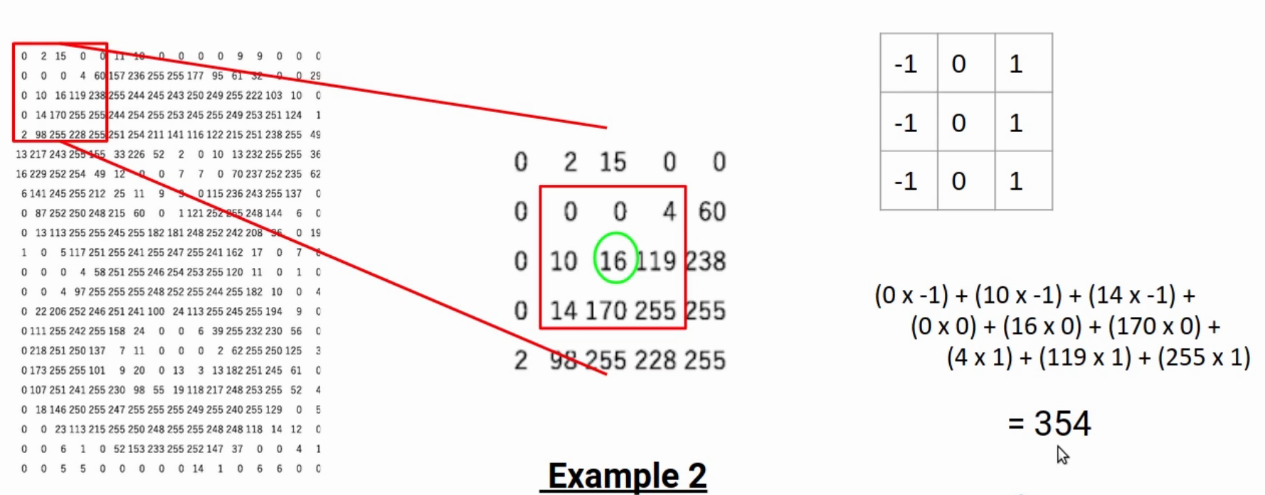In this example, the result is 354 which is significantly high.  Hence, we can say that the given pixel lies on edge.

### Filter/kernel

This matrix, that we use to calculate the difference is known as the filter or the kernel. This filter slides through the image to generate a new matric called a feature map. The values of the feature map tell, the particular pixel lies on the edge or not.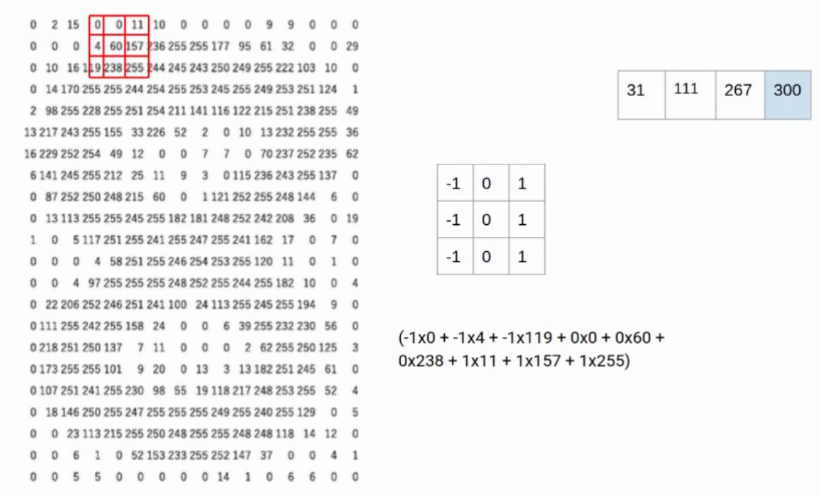The kernel we used in the above example is called the Prewitt kernel in the X-direction. Since it compares the values on the horizontal axis. Similarly, have a Prewitt kernel in Y-direction. Also, we have the Sobel kernel in X and Y directions.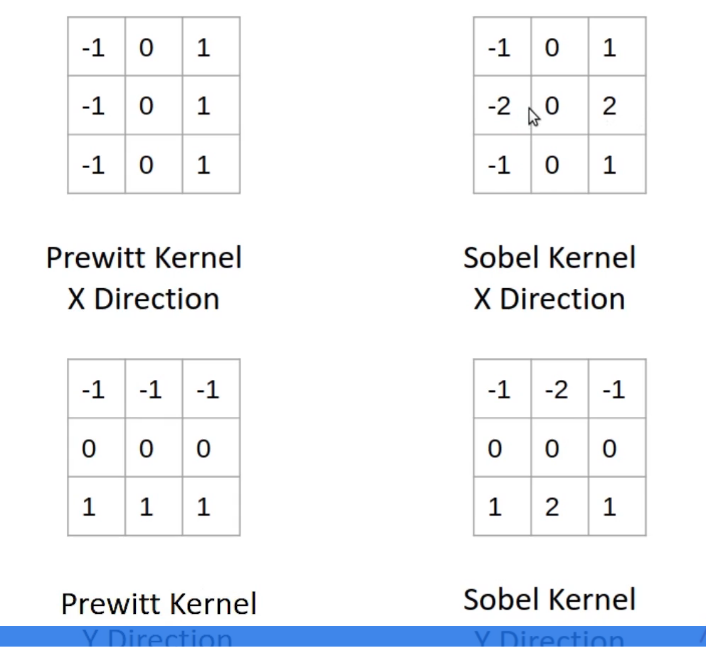In the case of Sobel kernels, higher importance is given to the pixel values right next to the target pixel.

## End Notes

To summarize, Pixels on the edge have a significant difference in values. We can compare neighboring pixel values to find the edge. Also, a matrix or a kernel is used to compare the values. The higher the difference between the right and left pixels, the target is closer to the edge. Similarly, Lower the difference- pixel is not at the edge.

If you are looking to kick start your Data Science Journey and want every topic under one roof, your search stops here. Check out Analytics Vidhya’s Certified AI & ML BlackBelt Plus Program

Let us know if you have any queries in the comments below regarding edge detection.